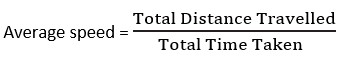## Motion and Time

I. Multiple Choice Questions (MCQs).
Q.1. The objects which change their positions with respect to other objects, are called in the state of
a) motion
b) movement
c) vibration
d) none of these
Q.2. When we talk about slow or fast motion, we in fact refer to its

a) Distance
b) displacement
c) speed
d) none of these

Q.3. Ancient people made time measuring devices such as sundial, hour-glass, water clock, etc., on the basis of
a) vibratory nature
b) periodic nature
c) oscillatory nature
d) none of these

Q.4. A special type of clock used to control the sequence of an event or process, is
a) clock having quartz crystal
b) stop watch
c) timer
d) none of these
Q.5. SI unit of speed is

a) km/h
b) m/min
c) m/s
d) all of these

II. Fill in the blanks by selecting appropriate words from the given list :
List : Unit, sundial, one-millionth, odometer, relative
Q.1. The state of rest and motion are ______________ terms.
Q.2. The speed of an object refers to the distance that object moves in ___________ time.
Q.3. A meter that measures the distance moved by a vehicle is known as ______________ .
Q.4. In the earliest time, measuring device that is based on the movement of sun in the sky was the ______________ .
Q.5. National Physical Laboratory, New Delhi has a clock that can measure a time period with an accuracy of ______________ of a second.

1- relative
2- Unit
3- odometer
4- sundial
5- one-millionth

III. Match the items of Column A with those of Column B.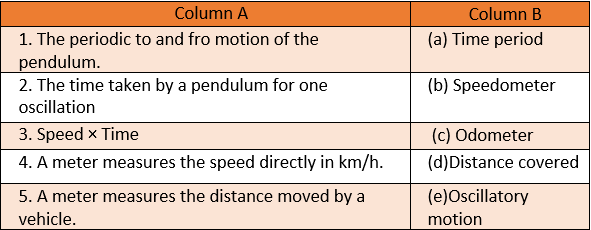IV. Write ‘T’ for the true and ‘F’ for the false statements.

Q.1. The pendulum completes one oscillation when its bob moves from one extreme position to the other.
Q.2. The time of sunrise and sunset were being used by earlier men to know the time.
Q.3. All clocks make use of some vibratory motion.
Q.4. Some clocks are also based on the oscillation of certain crystals like quartz.
Q.5. If the speed of a body moving along a straight line remains constant, its motion is said to be non-uniform.
Answer 1-F, 2-T, 3-F 4-T, 5-F
Q.1. How can you decide how fast an object is moving?
Answer: If an object covers a larger distance in a fixed interval of time than some other object, it is called faster and the other object is called slower.
Q.2. On which principle time measuring devices are based?
Answer: Periodic nature of a phenomenon.
Q.3. Name a special type of clock used to control the sequence of an event or process. Answer: A timer is a special type of clock used to control the sequence of an event or sequence or process.
Q.4. Which type of clock is used to measure time period of a pendulum?
Answer: Stop watch or clock, 5.
Q.5. Write the SI unit of speed.

Q.1. How can you say that the state of rest and motion are relative terms?
Answer: If you consider the motion of the earth, you will easily understand that the trees on earth, with reference to another object on earth is at rest, but with respect to other planet or star, it is in the motion along with the earth. Therefore, the state of rest and motion are relative terms.
Q.2. How does a clock having quartz crystal operate?
Answer: In quartz clock, fast vibrating crystal of quartz oscillates at a very precise rate and hence, measures time more accurately.

Q.3. What kind of discovery did lead to the development of pendulum clock?
Answer: Galileo was the first scientist who used a pendulum for the scientific purpose. According to legend, he watched a suspended Lamp swing back and forth in the cathedral of Pisa. He was surprised to find that his pulse beat same number of times during the interval in which the lamp completed one oscillation. Galilee experimented with various pendulums to verify his observation. He found that the time period does not depend on the arc of the swing. This discovery would lead to the development of pendulum clock.
Q.4. What do you understand by motion? How can you decide whether the trees or buildings are at rest or in motion? Give your opinion with suitable examples.
Answer: The objects which change their positions with respect to the objects at rest, are called in the state of motion.

A body relative to one object may be at rest, whereas relative to other object, it may be in motion. If you consider the motion of the earth (rotating or revolutionary), you will understand that the trees on earth, with reference to another object on earth is at rest, but with respect to other planet or star, it is in the motion along with the earth.

Q.5. How did ancient people use to measure time? Explain briefly.
Answer: Ancient people found out time by watching the natural events or phenomena. The time of sunrise and sunset were being used by earlier men to know the time. The time between one sunrise and one ancient people made devices such as Sundial, hour-glass [or sand clock], water clock, etc., to measure time.

Q.6. (a) A boy covers a distance of 2500 m in 1000 s with his bicycle. Calculate its speed in SI system.

(b) A car travels the distances of 60 km and 40 km in first and second hour respectively. Calculate its average speed in km/h.
Ans: a) Distance covered = 2500 m
Time 1000 s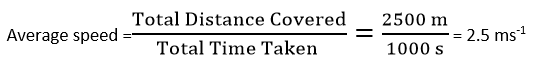b) The distance is 60 km hr–1 in 1 hour.
The distance is 40 km hr–1 in next hour.
Total distance travelled = 100 km
Total time taken = 2 hr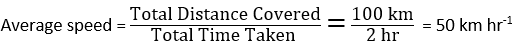Q.1. What do you mean by simple pendulum? Define all physical terms related to it.

Answer: A simple pendulum may be defined as a heavy point mass called bob ( a small brass or lead sphere) suspended by an inextensible thread from a fixed point and allowed to swing freely under the influence of gravity.

Terms describe a pendulum
(i) Length of the simple pendulum: The distance ‘l’ between the point of suspension and the centre of the bob.
(ii) Oscillation: One complete vibrational motion( to and fro motion). When the bob of the pendulum moves from one position and comes back to the same position, i.e., in the given figure A to C, C to B and B to A.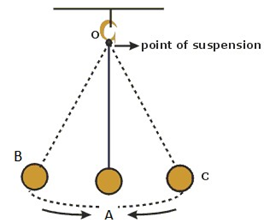(iii) Amplitude: The maximum displacement of the bob on either side from its mean position, i.e., from A to C or from A to B. AC is equal to AB.
(iv) Time Period: The time taken to complete one oscillation.

Q.2. How can we measure time? List some time measuring devices. Mention the units of time.
Answer: At present, time is measured by modern and advanced clocks and watches. Wristwatch, stopwatch, digital watch, pendulum clocks, etc. can be seen for measuring time.
The standard unit of time in seconds(s). The larger unit of time are minute, hour, day, month and year.

Q.3. (a) What do you mean by speed and average speed?
(b) What do you mean by uniform and non-uniform motions? Describe distance-time graph for uniform and non-uniform motions.

Answer: (a) Speed is the distance covered by an object in a unit time. It is the rate at which distance is covered with time, and is always measured in terms of a unit of distance divided by a unit of time,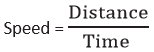Average Speed
No one vehicle moves with constant speed. They speed up, slow down and sometimes stop during the journey. Therefore, to determine how fast a vehicle moves, average speed is calculated.